Balbharti Maharashtra State Board 12th Chemistry Textbook Solutions Chapter 3 Ionic Equilibria Textbook Exercise Questions and Answers.

## Maharashtra State Board 12th Chemistry Solutions Chapter 3 Ionic Equilibria

1. Choose the most correct answer :

Question i.
The pH of 10-8 M of HCl is
(a) 8
(b) 7
(c) less than 7
(d) greater than 7
(c) less than 7

Question ii.
Which of the following solution will have pH value equal to 1.0?
(a) 50 mL of 0.1M HCl + 50mL of 0.1 M NaOH
(b) 60 mL of 0.1M HCl + 40mL of 0.1 M NaOH
(c) 20 mL of 0.1M HCl + 80mL of 0.1 M NaOH
(d) 75 mL of 0.2M HCl + 25mL of 0.2 M NaOH
(d) 75 mL of 0.2M HCl + 25mL of 0.2 M NaOH

Question iii.
Which of the following is a buffer solution ?
(a) CH3COONa + NaCl in water
(b) CH3COOH + HCl in water
(c) CH3COOH + CH3COONa in water
(d) HCl + NH4Cl in water
(c) CH3COOH + CH3COONa in water

Question iv.
The solubility product of a sparingly soluble salt AX is 5.2 x 10-13. Its solubility in mol dm-3 is
(a) 7.2 × 10-7
(b) 1.35 × 10-4
(c) 7.2 × 10-8
(d) 13.5 × 10-8
(a) 7.2 × 10-7Question v.
Blood in human body is highly buffered at pH of
(a) 7.4
(b) 7.0
(c) 6.9
(d) 8.1
(a) 7.4

Question vi.
The conjugate base of [Zn(H2O)4]2+ is
(a) [Zn(H2O)4]2+ NH3
(b) [Zn(H2O)3]2+
(c) [Zn(H2O)3OH]+
(d) [Zn(H2O)H]3+
(c) [Zn(H2O)3OH]+

Question vii.
For pH > 7 the hydronium ion concentration would be
(a) 10-7 M
(b) < 10-7 M
(c) > 10-7 M
(d) ≥ 10-7 M
(b) < 10-7 M

2. Answer the following in one sentence :

Question i.
Why cations are Lewis acids ?
Since cations are deficient of electrons they accept a pair of electrons, hence they are Lewis acids.

Question ii.
Why is KCl solution neutral to litmus?

1. Since KCl is a salt of strong base KOH and strong acid HCl, it does not undergo hydrolysis in its aqueous solution.
2. Due to strong acid and strong base, concentrations [H3O+] = [OH] and the solution is neutral.Question iii.
How are basic buffer solutions prepared?

1. Basic buffer solution is prepared by mixing aqueous solutions of a weak base like NH4OH and its salt of a strong acid like NH4Cl.
2. A weak base is selected according to the required pH or pOH of the solution and dissociation constant of the weak base.

Question iv.
Dissociation constant of acetic acid is 1.8 × 10-5. Calculate percent dissociation of acetic acid in 0.01 M solution.
Given : Ka = 1.8 x 10-5; C = 0.01 M
Percent dissociation = ?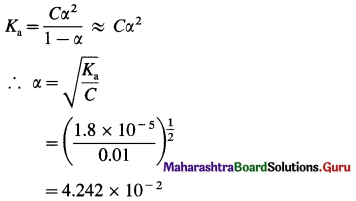∴ Percent dissociation = α × 100
= 4.242 × 10-2 × 102
= 4.242%
Percent dissociation = 4.242%

Question v.
Write one property of a buffer solution.
Properties (or advantages) of a buffer solution :

• The pH of a buffer solution is maintained appreciably constant.
• By addition of a small amount of an acid or a base pH does not change.
• On dilution with water, pH of the solution doesn’t change.

Question vi.
The pH of a solution is 6.06. Calculate its H+ ion concentration.

Question vii.
Calculate the pH of 0.01 M sulphuric acid.
Given : C = 0.01 M H2SO4, pH = ?
$$\mathrm{H}_{2} \mathrm{SO}_{4(\mathrm{aq})} \longrightarrow 2 \mathrm{H}_{(\mathrm{aq})}^{+}+\mathrm{SO}_{4(\mathrm{aq})}^{2-}$$
∴ [H3O+] = 2 × 0.01 = 0.02 M
PH = -log10 [H3O+]
= -log10 0.02
= –$$(\overline{2} .3010)$$
= 2 – 0.3010
= 1.6990
pH = 1.6990.

Question viii.
The dissociation of H2S is suppressed in the presence of HCl. Name the phenomenon.
The weak dibasic acid H2S is dissociated as follows :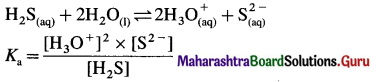When HCl is added, it increases the concentration of common ion H3O+.
$$\mathrm{HCl}_{(\mathrm{aq})}+\mathrm{H}_{2} \mathrm{O}_{(\mathrm{l})} \rightarrow \mathrm{H}_{3} \mathrm{O}_{(\mathrm{aq})}^{+}+\mathrm{Cl}_{(\mathrm{aq})}^{-}$$
Hence by Le Chaterlier’s principle, the equilibrium is shifted from right to left, suppressing the dissociation of weak electrolyte H2S.

Question ix.
Why is it necessary to add H2SO4 while preparing the solution of CuSO4?
CuSO4 is a salt of strong acid H2SO4 and weak base Cu(OH)2. CuSO4 in aqueous solution undergoes hydrolysis and forms a precipitate of Cu(OH)2 and solution becomes turbid.
CuSO4 + 2H2O ⇌ CU(OH)2↓ + H2SO4
OR
CuSO4 + 4H2O ⇌ Cu(OH)2 + 2H3O+ + $$\mathrm{SO}_{4}^{2-}$$
When H2SO4 is added, the hydrolysis equilibrium is shifted to left hand side and Cu(OH)2 dissolves giving clear solution.Question x.
Classify the following buffers into different types :
a. CH3COOH + CH3COONa
b. NH4OH + NH4Cl
c. Sodium benzoate + benzoic acid
d. Cu(OH)2 + CuCl2
(a) Acidic buffer (CH3COOH + CH3COONa)
(b) Basic buffer (NH4OH + NH4Cl)
(c) Acidic buffer (Sodium benzoate + benzoic acid)
(d) Basic buffer (Cu(OH)2 + CuCl2)
[Note : Cu(OH)2 being insoluble is not used to prepare a buffer solution.]

3. Answer the following in brief :

Question i.
What are acids and bases according to Arrhenius theory ?
According to Arrhenius theory :
Acid : It is a substance which contains hydrogen and on dissolving in water produces hydrogen ions (H+) E.g. HCl
$$\mathrm{HCl}_{(\mathrm{aq})} \rightleftharpoons \mathrm{H}_{(\mathrm{aq})}^{+}+\mathrm{Cl}_{(\mathrm{aq})}^{-}$$

Base : It is a substance which contains OH group and on dissolving in water produces hydroxyl ions (OH). E.g. NaOH
$$\mathrm{NaOH}_{(\mathrm{aq})} \rightleftharpoons \mathrm{Na}_{(\mathrm{aq})}^{+}+\mathrm{OH}_{(\mathrm{aq})}^{-}$$

Question ii.
What is meant by conjugate acid-base pair?
Conjugate acid-base pair : A pair of an acid and a base differing by a proton is called a conjugate acid-base pair.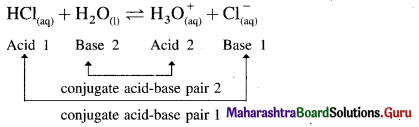Question iii.
Label the conjugate acid-base pair in the following reactions
a. HCl + H2O ⇌ H3O+ + Cl
b. $$\mathrm{CO}_{3}^{2-}$$ + H2O ⇌ OH + $$\mathrm{HCO}_{3}^{-}$$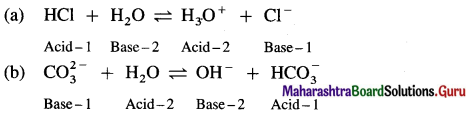Question iv.
Write a reaction in which water acts as a base.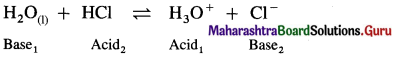Since water accepts a proton, it acts as a base.

Question v.
Ammonia serves as a Lewis base whereas AlCl3 is Lewis acid. Explain.

• Since ammonia molecule, NH3 has a lone pair of electrons to donate it acts as a Lewis base.
• AlCl3 is a molecule with incomplete octet hence it is electron deficient and acts as a Lewis acid.Question vi.
Acetic acid is 5% ionised in its decimolar solution. Calculate the dissociation constant of acid.
Given : C = 0.1 M; Dissociation = 5%, Ka=2 Percent dissociation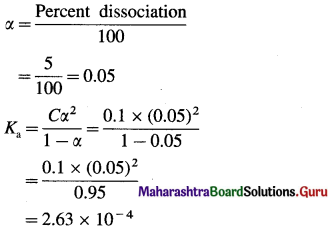Dissociation constant of acid = Ka = 2.63 × 10-4

Question vii.
Derive the relation pH + pOH = 14.
The ionic product of water, Kw is given by,
Kw = [H3O+] × [OH]
At 298 K, Kw = 1 × 10-14
∴ pKw = -log10Kw = log10 1 x 10-14 = 14
∵ [H3O+] × [OH] = 1 × 10-14
Taking logarithm to base 10 of both sides,
log10 [H3O+] + log10 [OH] = log10 1 x 10-14
Multiplying both the sides by -1,
-log10 [H3O+] -log10 [OH] = -log10 1 x 10-14
∵ pH = -log10 [H3O+]; pOH = -log10 [OH];
pKw = – log10 Kw
∴ pH + pOH = pKw
OR pH + pOH =14

Question viii.
Aqueous solution of sodium carbonate is alkaline whereas aqueous solution of ammonium chloride is acidic. Explain.
(A) (i) Sodium carbonate is a salt of weak acid and strong base.
(ii) In aqueous solution it undergoes hydrolysis.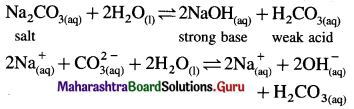(iii) Strong base dissociates completely while weak acid dissociates partially since [OH] > [H3O+], the solution is basic.

(B) (i) Ammonium chloride is a salt of strong acid and weak base.
(ii) In aqueous solution it undergoes hydrolysis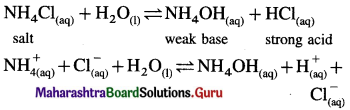(iii) Since [H+] or [H3O+ ] > [OH] the solution is acidic.

Question ix.
pH of a weak monobasic acid is 3.2 in its 0.02 M solution. Calculate its dissociation constant.
Given : pH = 3.2; C = 0.02 M; Ka = ?
pH = -log10 [H+]
∴ [H+] = Antilog – pH
= Antilog – 3.2
= Antilog $$\overline{4} .8$$
= 6.31 × 10-4M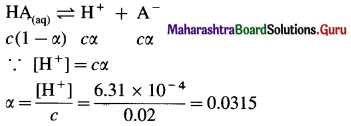Ka = cα2
= 0.02 × (0.0315)2
= 1.984 × 10-5
Dissociation constant = Ka = 1.984 × 10-5Question x.
In NaOH solution [OH] is 2.87 × 10-4. Calculate the pH of solution.
Given : [OH] = 2.87 × 10-4 M, pH = ?
pOH = -log10 [OH]
= -log10 2.87 × 10-4
= –$$(\overline{4} .4579)$$
= (4 – 0.4579)
= 3.5421
∵ pH + pOH = 14
∴ pH = 14 – pOH = 14 – 3.5421 = 10.4579
pH = 10.4579.

Question i.
Define degree of dissociation. Derive Ostwald’s dilution law for the CH3COOH.
(A) Degree of dissociation :
It is defined as a fraction of total number of moles of an electrolyte that dissociate into its ions at equilibrium.
It is denoted by a and represented by,
α = $$\frac{\text { number of moles dissociated }}{\text { total number of moles of an electrolyte }}$$
Or α = $$\frac{\text { Per cent dissociation }}{100}$$
∴ Per cent dissociation = α × 100

(B) Consider V dm3 of a solution containing one mole of CH3COOH. Then the concentration of acid is, C = $$\frac{1}{V}$$ mol dm3. Let α be the degree of dissociation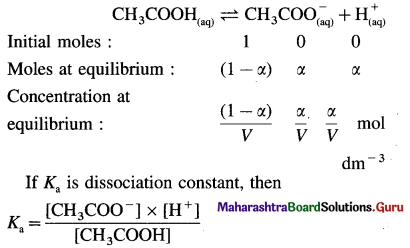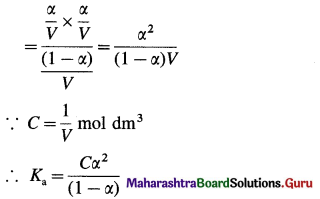This is Ostwald’s dilution law.

Question ii.
Define pH and pOH. Derive relationship between pH and pOH.
(1) pH : The negative logarithm, to the base 10, of the molar concentration of hydrogen ions, H+ is known as the pH of a solution.
pH = -log10 [H+]

(2) pOH : The negative logarithm, to the base 10, of the molar concentration of hydroxyl ions, OH is known as the pOH of a solution.
pOH = -log10 [OH]

Relationship between pH and pOH:
The ionic product of water, Kw is given by,
Kw = [H3O+] × [OH]
At 298 K, Kw = 1 × 10-14
∴ pKw = -log10Kw = log10 1 x 10-14 = 14
∵ [H3O+] × [OH] = 1 × 10-14
Taking logarithm to base 10 of both sides,
log10 [H3O+] + log10 [OH] = log10 1 x 10-14
Multiplying both the sides by -1,
-log10 [H3O+] – log10 [OH] = -log10 1 x 10-14
∵ pH = -log10 [H3O+]; pOH = -log10 [OH];
pKw = – log10 Kw
∴ pH + pOH = pKw
OR pH + pOH =14Question iii.
What is meant by hydrolysis ? A solution of CH3COONH4 is neutral. why ?
Hydrolysis : A reaction in which the cations or anions or both the ions of a salt react with water to produce acidity or basicity or sometimes neutrality is called hydrolysis.

A salt of weak acid and weak base for which Ka = Kb:
Consider hydrolysis of CH3COONH4.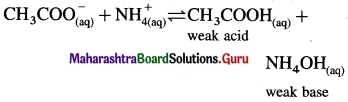Since Ka = Kb, the weak acid CH3COOH and weak base NH4OH dissociate to the same extent, hence, [H3O+] = [OH] and the solution reacts neutral after hydrolysis.

Question iv.
Dissociation of HCN is suppressed by the addition of HCl. Explain.
The weak acid HCN is dissociated as follows :
$$\mathrm{HCN}_{(\mathrm{aq})}+\mathrm{H}_{2} \mathrm{O}_{(\mathrm{l})} \rightleftharpoons \mathrm{H}_{3} \mathrm{O}_{(\mathrm{aq})}^{+}+\mathrm{CN}_{(\mathrm{aq})}^{-}$$
The dissociation constant Ka is represented as,
Ka = $$\frac{\left[\mathrm{H}_{3} \mathrm{O}^{+}\right] \times\left[\mathrm{CN}^{-}\right]}{[\mathrm{HCN}]}$$
When HCl is added, it increases the concentration of H3O+, hence in order to keep the ratio constant, then by Le Chatelier’s principle, the equilibrium is shifted from right to left, suppressing the dissociation of HCN.

Question v.
Derive the relationship between degree of dissociation and dissociation constant in weak electrolytes.
Expression of Ostwald’s dilution law in the case of a weak electrolyte : Consider the dissociation of a weak electrolyte BA. Let V dm3 of a solution contain one mole of the electrolyte. Then the concentration of a solution is, C = $$\frac{1}{V}$$mol dm-3. Let α be the degree of dissociation of the electrolyte.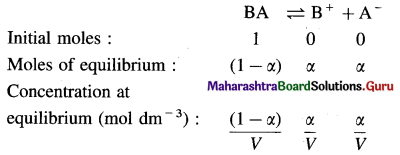Applying the law of mass action to this dissociation equilibrium, we have,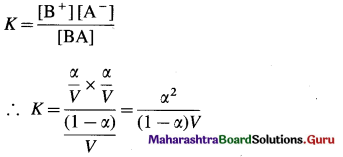As the electrolyte is weak, α is very small as compared to unity, ∴ (1 – α) ≈ 1.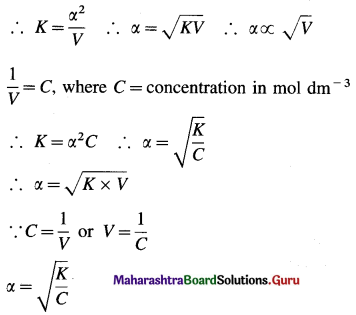This is the expression of Ostwald’s dilution law. Thus, the degree of dissociation of a weak electrolyte is directly proportional to the square root of the volume of the solution containing 1 mole of an electrolyte.

Question vi.
Sulfides of cation of group II are precipitated in acidic solution (H2S + HCl) whereas sulfides of cations of group IIIB are precipitated in ammoniacal solution of H2S. Comment on the relative values of solubility product of sulfides of these.
(1) In qualitative analysis, the cations of group II are precipitated as sulphides, namely HgS, CuS, PbS, etc., while cations of group IIIB are precipitated as sulphides, namely, CoS, NiS, ZnS.

(2) The sulphides of group II have extremely low solubility product (Ksp) about 10-29 to 10-53 while the sulphides of group IIIB have slightly higher Ksp values about 10-20 to 10-23.

(3) In group II, sulphides are precipitated by adding H2S in acidic solution while in IIIB group they are precipitated in a basic solution like ammonical solution.

(4) In acidic medium due to common ion H+, H2S is dissociated to very less extent but gives sufficient S2- ion to exceed solubility product of group II sulphides of cations and precipitate them.
$$\mathrm{HCl}_{(\mathrm{aq})} \longrightarrow \mathrm{H}_{(\mathrm{aq})}^{+}+\mathrm{Cl}_{(\mathrm{aq})}^{-} ; \mathrm{H}_{2} \mathrm{~S}_{(\mathrm{aq})} \rightleftharpoons 2 \mathrm{H}_{(\mathrm{aq})}^{+}+\mathrm{S}_{(\mathrm{aq})}^{2-}$$

(5) In basic medium, H+ from H2S are removed by OH in solution, or by NH4OH, increasing the dissociation of H2S and concentration of S2-, so that IP > Ksp.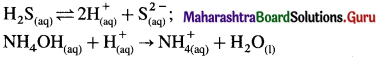(6) Therefore group II cations are precipitated in an acidic medium while cations of group IIIB are precipitated in ammonical solution.

Question vii.
Solubility of a sparingly soluble salt get affected in presence of a soluble salt having one common ion. Explain.
Consider the solubility equilibrium of a sparingly soluble salt, AgCl.
$$\mathrm{AgCl}_{(\mathrm{s})} \rightleftharpoons \mathrm{Ag}_{(\mathrm{aq})}^{+}+\mathrm{Cl}_{(\mathrm{aq})}^{-}$$
The solubility product, Ksp is given by,
Ksp = [Ag+] × [Cl]
Consider addition of a strong electrolyte AgNO3 with a common ion Ag+.
$$\mathrm{AgNO}_{3(\mathrm{aq})} \longrightarrow \mathrm{Ag}_{(\mathrm{aq})}^{+}+\mathrm{NO}_{3(\mathrm{aq})}^{-}$$
The concentration Ag+ in the solution is increased, hence by Le Chatelier’s principle the equilibrium of AgCl is shifted to left hand side since the value of Ksp is constant.
Thus in the presence of a common ion, the solubility of a sparingly soluble salt is suppressed.Question viii.
The pH of rain water collected in a certain region of Maharashtra on particular day was 5.1. Calculate the H3O+ ion concentration of the rain water and its percent dissociation.
Given : pH = 5.1, [H3O+] = ?
PH = -log10 [H3O+]
∴ log10 [H3O+] = -pH
∴ [H3O+] = Antilog – pH
= Antilog – 5.1
= Antilog $$\overline{6} .9$$
= 7.943 × 10-6 M
(H3O+ in rainwater is due to dissolved gases, CO2, SO2, etc. forming acids which dissociate giving H3O+ and acidity to rainwater.)
[H3O+] = 7.943 × 10-4 M

Question ix.
Explain the relation between ionic product and solubility product to predict whether a precipitate will form when two solutions are mixed?
If ionic product and solubility product are indicated by IP and Ksp respectively then,
(I) When IP = Ksp, the solution is saturated.
(II) When IP > Ksp, the solution is supersaturated and hence precipitation will occur, when two solutions are mixed.
(Ill) When IP < Ksp, the solution is unsaturated and precipitation will not occur, when two solutions are mixed.

12th Chemistry Digest Chapter 3 Ionic Equilibria Intext Questions and Answers

Use your brain power (Textbook Page No. 47)

Question 1.
Which of the following is a strong electrolyte ?
HF, AgCl, CuSO4, CH3COONH4, H3PO4.
CH3COONH4 is a strong electrolyte since in aqueous solution it dissociates completely. Sparingly soluble salts AgCl, CuSO4 are also strong electrolytes.

Use your brain power (Textbook Page No. 49)

Question 1.
All Bronsted bases are also Lewis bases, but all Bronsted acids are not Lewis acids. Explain.
NH3 is a Bronsted base since it can accept a proton while it is also a Lewis base since it has a lone pair of electrons to donate.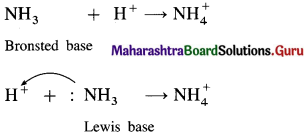(2) HCl is a Bronsted acid since it can donate a proton but it is not a Lewis acid since it can’t accept a pair of electrons.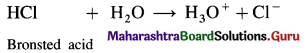Use your brain power (Textbook Page No. 53)

Question 1.
Suppose that pH of monobasic and dibasic acid is the same. Does this mean that the molar concentrations of both acids are identical ?
Even if monobasic acid and dibasic acid give same pH, their molar concentrations are different. One mole of monobasic acid like HCl gives 1 mol of H+ while one mole of dibasic acid gives 2 mol of H+ in solution. Hence the concentration of dibasic acid will be half of the concentration of monobasic acid. For example, for same pH. [Monobasic acid] = [Dibasic acid]/2Question 2.
How does pH of pure water vary with temperature ? Explain.
Since the increase in temperature, increases the dissociation of water, its pH decreases.

Can you tell ? (Textbook Page No. 54)

Question 1.
Why (i) an aqueous solution of NH4Cl is acidic.
(ii) while that of HCOOK basic ?
(i) (i) Ammonium chloride is a salt of strong acid and weak base.
(ii) In aqueous solution it undergoes hydrolysis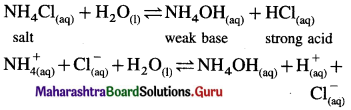(iii) Since [H+] or [H3O+] > [OH] the solution is acidic.

(ii) HCOOK is a salt of weak acid HCOOH and strong base KOH. In aqueous solution it undergoes hydrolysis giving weak acid and strong base KOH which dissociates completely,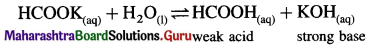∴ [OH] > [H3O+], and the solution reacts basic.

Can you think ? (Textbook Page No. 56)

Question 1.
Home made jams and jellies without any added chemical preservative additives spoil in a few days whereas commercial jams and jellies have a long shelf life. Explain. What role does added sodium benzoate play ?
Sodium benzoate added to jams and jellies in commercial products maintains the pH constant and acts as a preservative. Hence jams and jellies are not spoiled for a very long time unlike homemade products.

Can you tell ? (Textbook Page No. 56)

Question 1.
It is enough to add a few mL of a buffer solution to maintain its pH. Which property of buffer is used here ?
The important property of reserve acidity and reserve basicity of a buffer solution is used to maintain constant pH. Weak acid or weak base along with ions (cations or anions) from salt react with excess of added acid (H+) or base [OH] and maintain pH constant.Use your brain power (Textbook Page No. 59)

Question 1.
What is the relationship between molar solubility and solubility product for salts given below : (i) Ag2CrO4 (ii) Ca3(PO4)2 (iii) Cr(OH)3.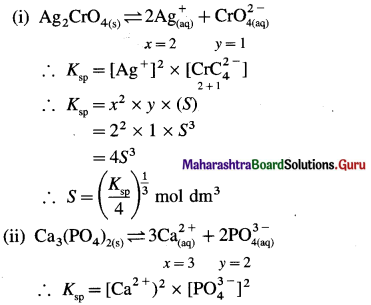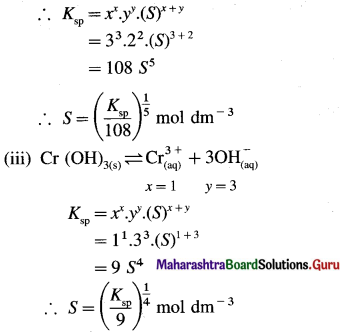Can you tell ? (Textbook Page No. 60)

Question 1.
How is the ionization of NH4OH suppressed by addition of NH4Cl to the solution of NH4OH ?
$$\mathrm{NH}_{4} \mathrm{OH}_{(\mathrm{aq})} \rightleftharpoons \mathrm{NH}_{4(\mathrm{aq})}^{+}+\mathrm{OH}_{(\mathrm{aq})}^{-}$$
Kb = $$\frac{\left[\mathrm{NH}^{4+}\right] \times\left[\mathrm{OH}^{-}\right]}{\left[\mathrm{NH}_{4} \mathrm{OH}\right]}$$
$$\mathrm{NH}_{4} \mathrm{Cl}_{(\mathrm{aq})} \longrightarrow \mathrm{NH}_{4(\mathrm{aq})}^{+}+\mathrm{Cl}_{(\mathrm{aq})}^{-}$$
Due to common ion $$\mathrm{NH}_{4}^{+}$$, by Le Chatelier’s principle, the equilibrium is shifted from right to left, suppressing the ionisation of NH4OH.